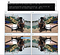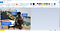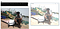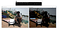# Understanding Computer Vision: Part 3

1. Basic Image Processing
a. Rotation
b. Resizing
c. Flipping
e. Cropping
f. Image Arithmetic

## Rotation

The cv2.getRotationMatrix2D function takes three arguments. The first argument is the point in which we want to rotate the image about (in this case, the center of the image). We then specify \theta, the number of (counter-clockwise) degrees we are going to rotate the image by. In this case, we are going to rotate the image 45 degrees. The last argument is the scale of the image. We haven’t discussed resizing an image yet, but here you can specify a floating point value, where 1.0 means the same, original dimensions of the image are used.

However, if you specified a value of 2.0, the image would be doubled in size. Similarly, a value of 0.5 halve the size of the image.

Once we have our rotation matrix M from the cv2.getRotationMatrix2D function, we can apply the rotation to our image using the cv2.warpAffine method.

#Rotate by 45 degree

import cv2
import argparse
import numpy as np

apr = argparse.ArgumentParser()
apr.add_argument(“-i”, “ — image”, required=True, help=”Path to the image”)
args = vars(apr.parse_args())

cv2.imshow(“Original Image”,image)
print(f’(Height,Width,Depth) of the Original image is: {image.shape}’)

# Rotation — find centre of the image by using the already known formula
height = image.shape
width = image.shape
(cX,cY) = (width//2,height//2)

#rotate our image by 45 degree

M = cv2.getRotationMatrix2D((cX,cY),-45,1.0)
rotated = cv2.warpAffine(image, M, (width, height))
cv2.imshow(“Rotated by 45 Degrees”, rotated)
cv2.waitKey(0)
#
rotate our image around an arbitrary point rather than the center
#M = cv2.getRotationMatrix2D((cX — 50, cY — 50), 45, 1.0)

The first argument to this function is the image we want to rotate. We then specify our rotation matrix M along with the output dimensions (width and height) of our image.Shows our image rotated by 45 degrees. Similarity we can change the degree of rotation by changing the second parameter of cv2.gerRotationMatrix2D to 90 degrees.

M = cv2.getRotationMatrix2D((cX,cY),-90,1.0)

## Resizing

Photo

we need to keep in mind aspect ratio so the image does not look skewed or distorted — therefore, we calculate the ratio of the new image to the old image. Let’s make our new image have a width of 250 pixels

After we compute our ratio, we can compute the new dimensions of the image . Again, the width of the new image will be 250 pixels. The height is then computed by multiplying the old height by our ratio and converting it to an integer.

By performing this operation we are able to preserve the original aspect ratio of the image

#Resize

import cv2
import argparse
import numpy as np

apr = argparse.ArgumentParser()
apr.add_argument(“-i”, “ — image”, required=True, help=”Path to the image”)
args = vars(apr.parse_args())

cv2.imshow(“Original Image”,image)
print(f’(Height,Width,Depth) of the Original image is: {image.shape}’)

r = 250.0 / image.shape
#
(width, new height )
dim = (250,int(image.shape *r))
# cv2.resize(input image, new dimension ,interpolation )
resized = cv2.resize(image,dim,interpolation = cv2.INTER_AREA)
#
The last parameter is our interpolation method, which is the algorithm working behind the scenes to handle how the actual image is resized.
print(f’(Height,Width,Depth) of the resized image is: {resized.shape}’)
cv2.imshow(“resized”, resized)
cv2.waitKey(0)

Aspect Ratio = Width / Height

If we aren’t mindful of the aspect ratio, our resizing will return results that look distorted.

## FlippingFIG 3.3 FLIP THE IMAGE BY HORIZONTAL,VERTICAL AND BY BOTH AXES

#FLIP HORIZONTAL,VERTICAL AND BY BOTH AXES

import cv2
import argparse
import numpy as np

apr = argparse.ArgumentParser()
apr.add_argument(“-i”, “ — image”, required=True, help=”Path to the image”)
args = vars(apr.parse_args())

cv2.imshow(“Original Image”,image)

print(f’(Height,Width,Depth) of the Original image is: {image.shape}’)

#Horizontally
flipp_h = cv2.flip(image,1)
#
Vertically
flipp_v= cv2.flip(image,0)
#
flip along both axes
flipp_b = cv2.flip(image,-1)
cv2.imshow(‘Horizontal Flip’, flipp_h)
cv2.imshow(‘Vertical Flip ‘, flipp_v)
cv2.imshow(‘flipped by both axes’, flipp_b)
cv2.waitKey(0)

## Cropping

Cropping is something that we have already done above using NumPy Slicing. Here we are going to use MSPaint for one last time to extract the co-ordinates of our region of interest.FIG 3.5 HOW TO PICK x,y CO-ORDINATES OF REGIONS USING MS-PAINT

Lets try to crop my face from the original image using python code.

**syntax => image[y1:y2 , x1:x2] ||| MS Paint order — (x1:y1 , x2,y2)

#CROP FACE FROM IMAGE
import cv2
import argparse
import numpy as np

apr = argparse.ArgumentParser()
apr.add_argument(“-i”, “ — image”, required=True, help=”Path to the image”)
args = vars(apr.parse_args())

cv2.imshow(“Original Image”,image)

print(f’(Height,Width,Depth) of the Original image is: {image.shape}’)
#
syntax => image[y1:y2 , x1:x2] ||| MS Paint order — (x1:y1 , x2,y2)
crop = image[71:220,382:527]

cv2.imshow(‘crop’,crop)
cv2.waitKey(0)

Going forward we will develop deep learning algorithms that can automatically detect the object’s of interest in an image like face,vehicle number plate, humans, cars,animals in a data driven way. The deep learning algorithms can automatically localize the objects in the image and provide us the x,y co-ordinates instead of us having to use MS Paint to find the co-ordinates. By that time we will make us of the above crop function to extract the objects of interest.

Say for example if we manage to locate the vehicle number plate in a vechicle using deep learning algorithm, we may be in a position to crop and extract the number plates to create a datasets. We can make use of this crop function by that time.

## Image Arithmetic

You can add two images by OpenCV function, cv2.add() or simply by numpy operation, res = img1 + img2. Both images should be of same depth and type, or second image can just be a scalar value.

However, there is a difference between OpenCV addition and Numpy addition. OpenCV addition is a saturated operation while Numpy addition is a modulo operation.

>>> x = np.uint8()>>> y = np.uint8()# OpenCV addition>>> print cv2.add(x,y) # 250+10 = 260 => 255[]#NumPy addition >>> print x+y          # 250+10 = 260 % 256 = 4

In the above example, when working with images, we need to keep in mind the limits of our color space and data type.For example, RGB images have pixels that fall within the range [0, 255]. What happens if we are examining a pixel with intensity 250 and we try to add 10 to it ? as we did in the above example.

Under normal arithmetic rules, we would end up with a value of 260. However, since RGB images are represented as 8-bit unsigned integers, 260 is not a valid value.

So what should happen? Should we perform a check of some sorts to ensure no pixel falls outside the range of [0, 255], thus clipping all pixels to have a minimum value of 0 and a maximum value of 255?

OpenCV will perform clipping and ensure pixel values never fall outside the range [0, 255].

So be sure to keep in mind that there is a difference between OpenCV and NumPy addition. NumPy will perform modulus arithmetic and “wrap around.” OpenCV, on the other hand, will perform clipping and ensure pixel values never fall outside the range [0, 255].FIG 3.6 IMAGE AFTER ADDING A VALUE OF 100 TO EVERY PIXEL VALUE. NOTICE HOW THE IMAGE NOW LOOKS WASHED OUT.

#ADDING A VALUE OF 100 TO EVERY PIXEL VALUE. NOTICE HOW THE IMAGE NOW LOOKS WASHED OUT.
import cv2
import argparse
import numpy as np

apr = argparse.ArgumentParser()
apr.add_argument(“-i”, “ — image”, required=True, help=”Path to the image”)
args = vars(apr.parse_args())

cv2.imshow(“Original Image”,image)

M= np.ones(image.shape, dtype = “uint8”) * 100
cv2.waitKey(0)

Notice how the image looks more “washed out” and is substantially brighter than the original. This is because we are increasing the pixel intensities by adding 100 to them and pushing them towards brighter colors.

However, we can add two separate images also by passing the two image object as parameters to cv2.add function.FIG 3.7 IMAGE AFTER SUBTRACTING A VALUE OF 100 FROM EVERY PIXEL VALUE. NOTICE HOW THE IMAGE NOW LOOKS CONSIDERABLY DARKER.

#SUBTRACTING A VALUE OF 100 TO EVERY PIXEL VALUE. NOTICE HOW THE IMAGE NOW LOOKS DARKER.
import cv2
import argparse
import numpy as np

apr = argparse.ArgumentParser()
apr.add_argument(“-i”, “ — image”, required=True, help=”Path to the image”)
args = vars(apr.parse_args())

cv2.imshow(“Original Image”,image)

M= np.ones(image.shape, dtype = “uint8”) * 100
sub = cv2.subtract(image, M)
cv2.imshow(“Subtracted”, sub)
cv2.waitKey(0)

Notice how darker the image look to that of the original image.Pixels that were once white now look gray. This is because we are subtracting 50 from the pixels and pushing them towards the darker regions of the RGB color space.